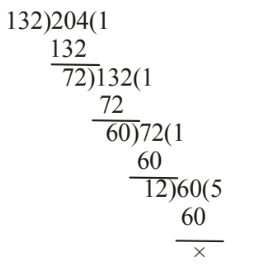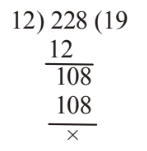## LCM and HCF

#### LCM and HCF

1. Three plots having an area of 132, 204 and 228 square metres respectively are to be subdivided into equal vegetable beds. If the breadth of a bed is 3 metres, find the maximum length that a bed can have.

1. The maximum area a bed can have will be the greatest divisor of three plots.
we find the HCF of 132, 204 and 228
Now, HCF of 132 and 204The HCF of 132 and 204 = 12
The required HCF = HCF of 12 and 228.Hence, the greatest area of the equalised bed = 12 sq.metres

##### Correct Option: B

The maximum area a bed can have will be the greatest divisor of three plots.
we find the HCF of 132, 204 and 228
Now, HCF of 132 and 204The HCF of 132 and 204 = 12
The required HCF = HCF of 12 and 228.Hence, the greatest area of the equalised bed = 12 sq.metres

 ∴ Maximum length of the bed = Area = 12 = 4 metres Breadth 3

1. The sum of two numbers is 1215 and their HCF is 81. How many such pairs of numbers can be formed?

1. Let the numbers be 81p and 81q , where p and q are co-prime.
∴ 81p + 81q = 1215

 ⇒ p + q = 1215 = 15 81

##### Correct Option: B

Let the numbers be 81p and 81q , where p and q are co-prime.
∴ 81p + 81q = 1215

 ⇒ p + q = 1215 = 15 81

Possible pairs = (1 , 14), (2 , 13), (4 , 11), (7 , 8)
Hence , required answer is 4.

1. The number of pair of positive integers whose sum is 99 and HCF is 9 is

1. Let the numbers be 9x and 9y where x and y are prime to each other.
According to the question,
9x + 9y = 99
⇒ 9(x + y) = 99
⇒ x + y = 11
Possible pairs = (1, 10) (2, 9), (3, 8), (4, 7), (5, 6)

##### Correct Option: D

Let the numbers be 9x and 9y where x and y are prime to each other.
According to the question,
9x + 9y = 99
⇒ 9(x + y) = 99
⇒ x + y = 11
Possible pairs = (1, 10) (2, 9), (3, 8), (4, 7), (5, 6)

1. The HCF (GCD) of a, b is 12, a, b are positive integers and a > b > 12. The smallest values of (a, b) are respectively

1. HCF of a and b = 12
∴ Numbers = 12p and 12q , where p and q are prime to each other.
∴ a > b > 12

##### Correct Option: D

HCF of a and b = 12
∴ Numbers = 12p and 12q , where p and q are prime to each other.
∴ a > b > 12
∴ a = 36 ; b = 24

1. A fraction becomes 1/6 when 4 is subtracted from its numerator and 1 is added to its denominator. If 2 and 1 are respectively added to its numerator and the denominator, it becomes 1/3 Then, the LCM of the numerator and denominator of the said fraction, must be

1.  Let the original fraction be p . q

 ∴ p - 4 = 1 q + 1 6

⇒ 6p – 24 = q + 1
⇒ 6p – q = 25 .......(i)
Again,
 ∴ p + 2 = 1 q + 1 3

##### Correct Option: D

 Let the original fraction be p . q

 ∴ p - 4 = 1 q + 1 6

⇒ 6p – 24 = q + 1
⇒ 6p – q = 25 .......(i)
Again,
 ∴ p + 2 = 1 q + 1 3

⇒ 3p + 6 = q + 1
⇒ 3p – q = –5 .......(ii)
By equation (i) – (ii),
6p – q – 3p + q = 25 + 5
⇒ 3p = 30 ⇒ p = 10
From equation (i),
60 – q = 25 ⇒ q = 35
LCM of 10 and 35 = 70